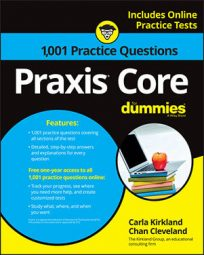##### Praxis Core: 1,001 Practice Questions For DummiesEven when two shapes look identical, they may not be. For example, on the Praxis Core exam, you may encounter a question where two shapes look the same, but you're told they are "similar." What does this mean? What if they're labeled "congruent"?

If you're not sure, the following practice questions (and their explanations) should refresh your memory. In the first question, you're shown two parallelograms that are similar, and you have to find their scale factor. The second question shows you two congruent triangles, and asks you to select all of the true statements about them.

## Practice questions

Refer to the following figure for the first question.

1. The two parallelograms in the following diagram are similar. Line BD corresponds to line FH, and line AB corresponds to EF. What is the measure of line EF?

The two triangles in the following diagram are congruent.

2. Which of these statements is true? Select all that apply.

A. All pairs of corresponding angles are congruent. B. All pairs of corresponding sides are congruent. C. All corresponding parts are congruent. D. The two triangles' perimeters are equal. E. All pairs of corresponding angles are congruent, but none of the pairs of corresponding sides are necessarily congruent.

1. The correct answer is Choice (E).

You can set up a proportion with ratios of corresponding side measures. Use a variable to represent the unknown measure:

The side measure in question is 6 m.

2. The correct answers are Choices (A, B, C, and D).

If two triangles are congruent, they're identical; they're essentially the same triangle in two different places. By definition, all pairs of corresponding parts between two congruent triangles are congruent. That means their corresponding sides are congruent in every case, and their corresponding angles are congruent in every case. Because their corresponding sides are congruent, the sums of their sides are the same, so their perimeters are equal.

Choice (E) isn't true, because all pairs of corresponding sides are congruent in all cases of congruent triangles. Choice (E) is true of similar triangles but not congruent triangles.